Tutorials
data analysis

Learn how to apply operations like add, subtract, divide, multiply, and a lot more in Google Spreadsheets with the help of an actual dataset.

Note: If you would like to learn about conditional formatting in spreadsheets or pivot tables in spreadsheets or graphs in spreadsheets, please feel free to check out all the tutorials. All of these topics will provide great insight into visualizing, analyzing and manipulating your data in Google Spreadsheets.

Introduction

You have all done basic maths in your primary school and continue to do so in your day-to-day life may be by using a calculator, or if it's just adding two small numbers, then you might calculate the result manually in your head.

However, what if instead of adding/dividing two numbers you have to perform these mathematical operations on a series of rows or cells or columns? Using the calculator will not be a way to go. Hence, you need Google Spreadsheets.

Google spreadsheets provide you with a useful feature called Function that not only has some basic mathematical operations but more advanced operations that can be very useful for you. Some of the operations involve add, subtract, multiply, divide, square root, exponent, finding averages, etc. and you can even build your custom formulas.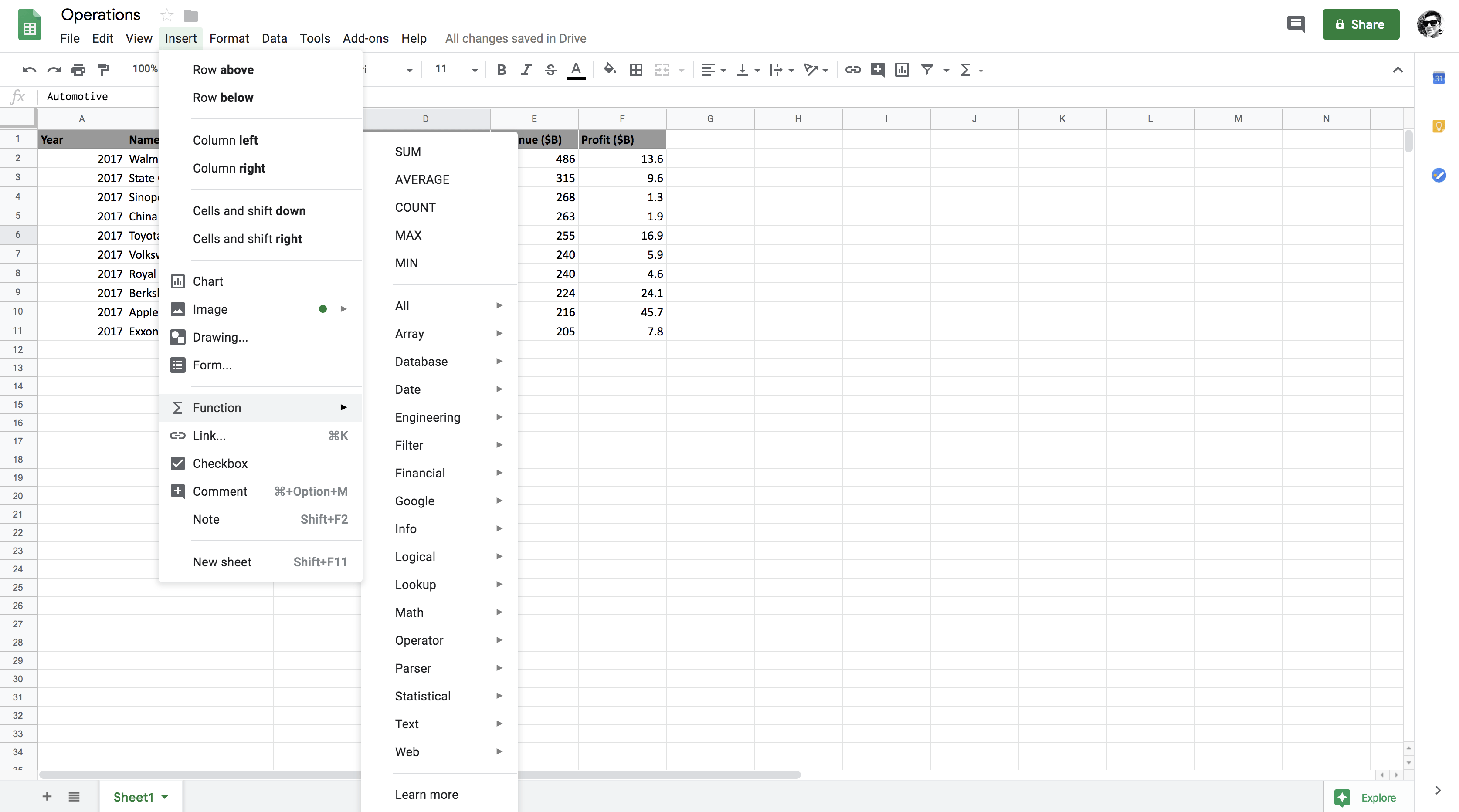As you can see under the Insert tab, the Function feature has numerous operations that can be used within the Google Spreadsheet. In today's tutorial, you will be using some of these operations. Function is built-in spreadsheets which offers a vast variety of formulas.

Google Spreadsheets follows PEMDAS as the order of operation. Order of operations means the order in which the mathematical process will occur. To simplify it a bit, if a formula has a combination of addition, multiplication, and division, all three operations will follow a particular order in which they will be performed.

PEMDAS stands for:

• Parentheses (Brackets)
• Exponents
• Multiplication
• Division
• Subtraction

Now, let's look at the dataset that you will be using in this tutorial.

You will start with a dataset that is organized into a row and column format. This particular dataset shows the top 10 companies in the Fortune Global 500 in 2017 which is based on highest gross revenues column.

Though this dataset has many attributes, for this tutorial, you will be primarily using only the Revenue and Profit numerical attributes.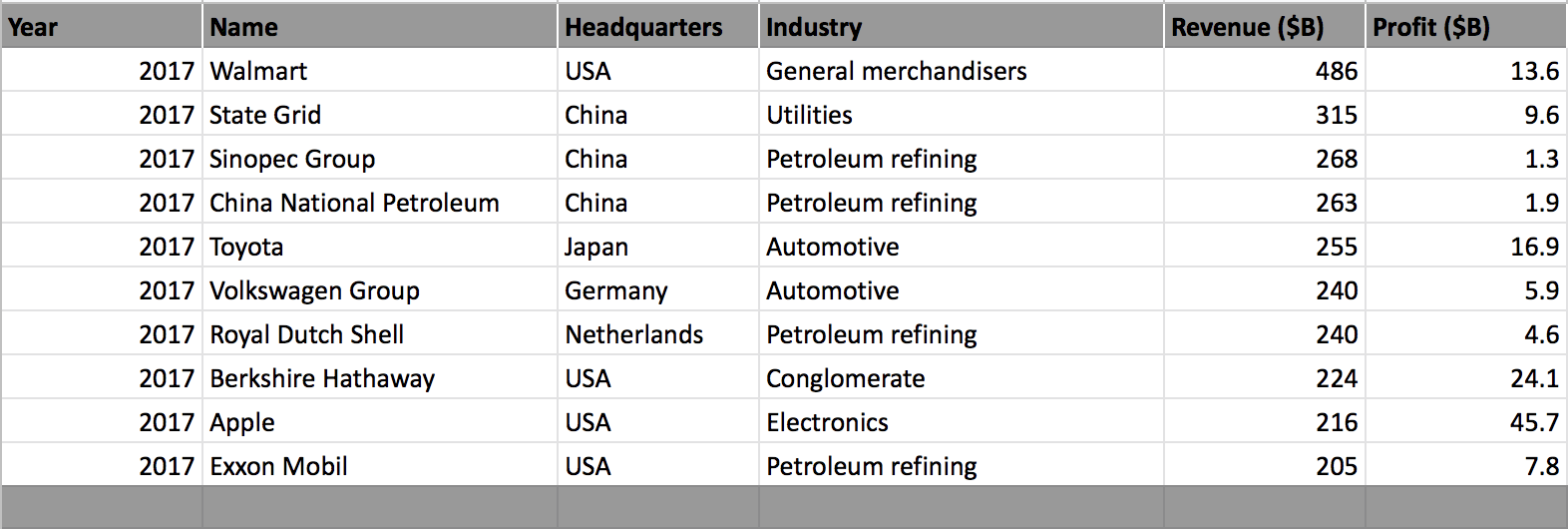You will learn to apply various operations on the above dataset and also learn about some outliers that you need to keep in mind while applying the mathematical operations.

Note: Please make sure you have a valid Gmail account, once you have an account all you need to do is click on this link and create a new blank spreadsheet.

The data is available here.

Mathematical Operations

• Add sums up two numbers and returns the final value. ADD(value1, value2) where value1 and value2 are the two addend. For example: =ADD(2,3) will return a value 5.

Note: All the formulas should have an = equal operator as a prefix, only then google spreadsheet will consider it as a valid formula.

Let's select one value each from Revenue and Profit and apply the ADD function to it. You will choose a value from cell E2 and F2 and pass these two values as a parameter to the ADD function.

As shown in the below figure, =ADD(E2, F2) selects cell E2 and F2 which will be summed.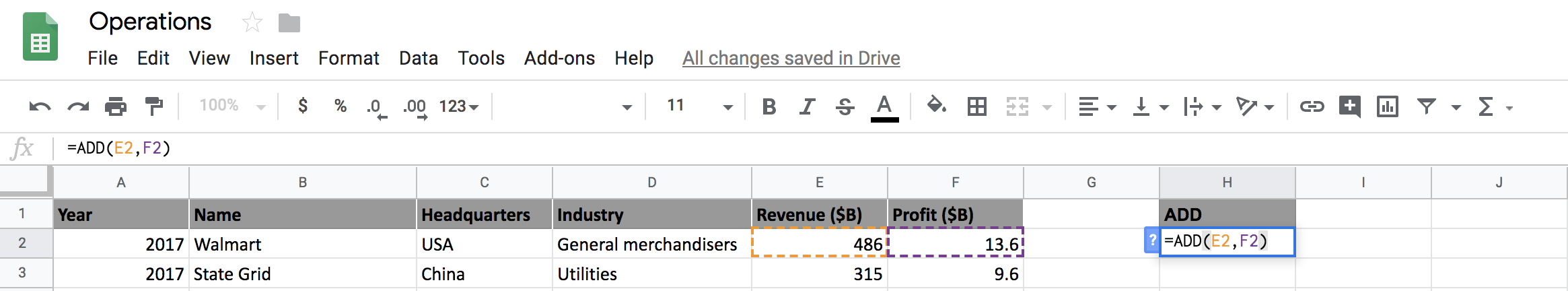In the below figure, you can see that the addition of cell E2 and F2 is 500. You can also observe that, ADD operation can be performed manually by passing the two cells E2 and F2 separated with a + (plus) operator which also gives the same result as the ADD function.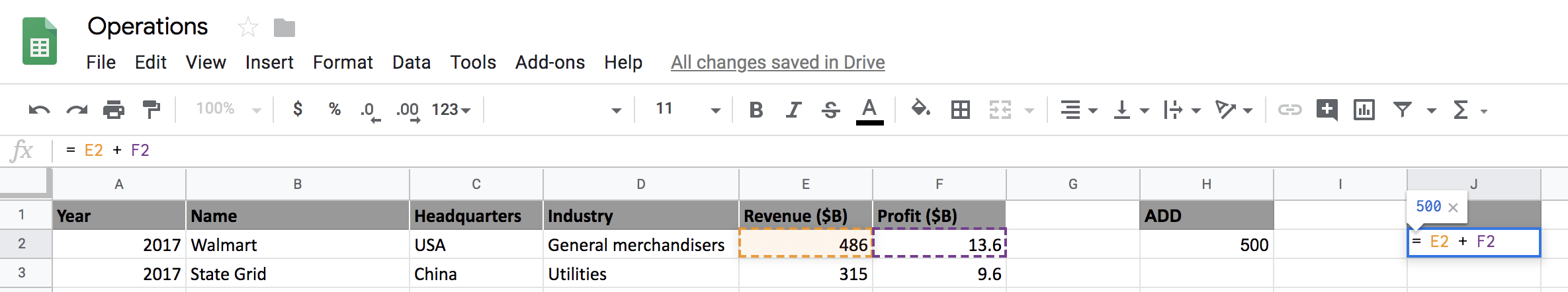Next, you will perform the SUM operation and also find out how it is different from the ADD operation.

• SUM function returns the sum of a series of numbers and/or cells. It can be written in many ways like SUM(value1, [value2, ...]) or SUM([value1, ...], value2) or SUM([value1, ...],[value2, ...]). All three perform different sum operations over different cell/cells.

The main difference between ADD and SUM operation is that SUM operation can be performed over multiple cells of a column whereas an ADD operation can only be performed on a scalar value.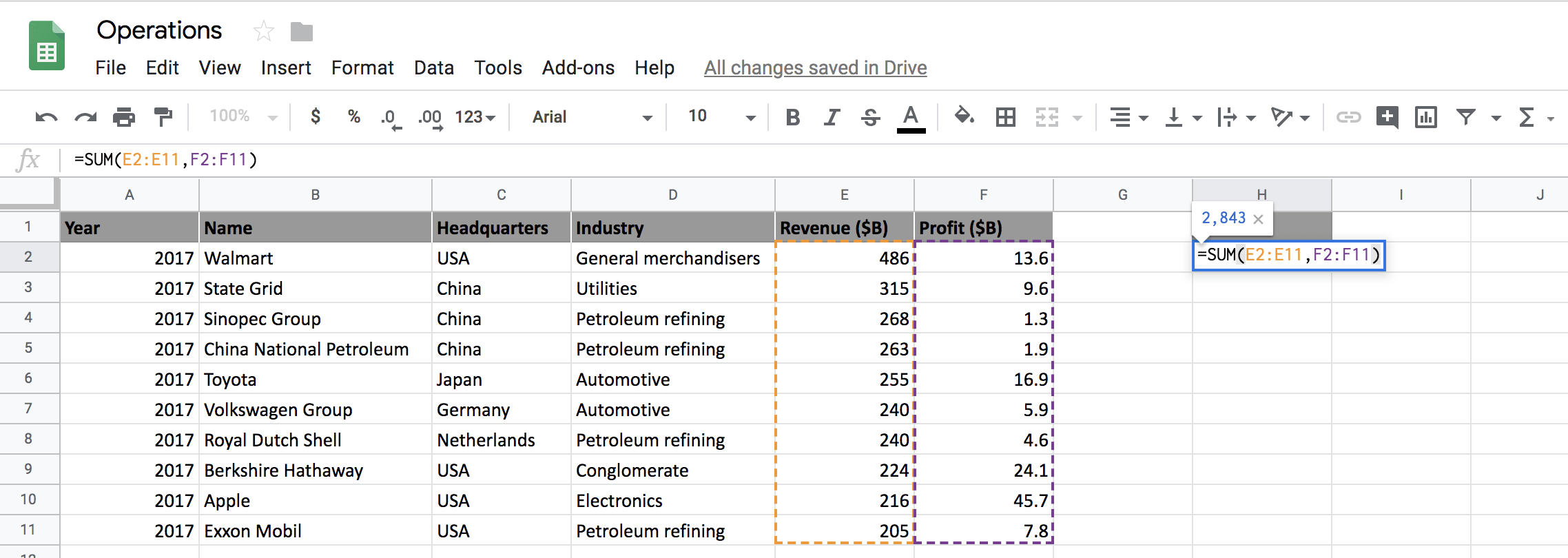As shown in the above figure, you can select two complete columns and sum them up using the SUM function.

Similarly, you can select only a subset of the two columns and sum them as shown in the figure below.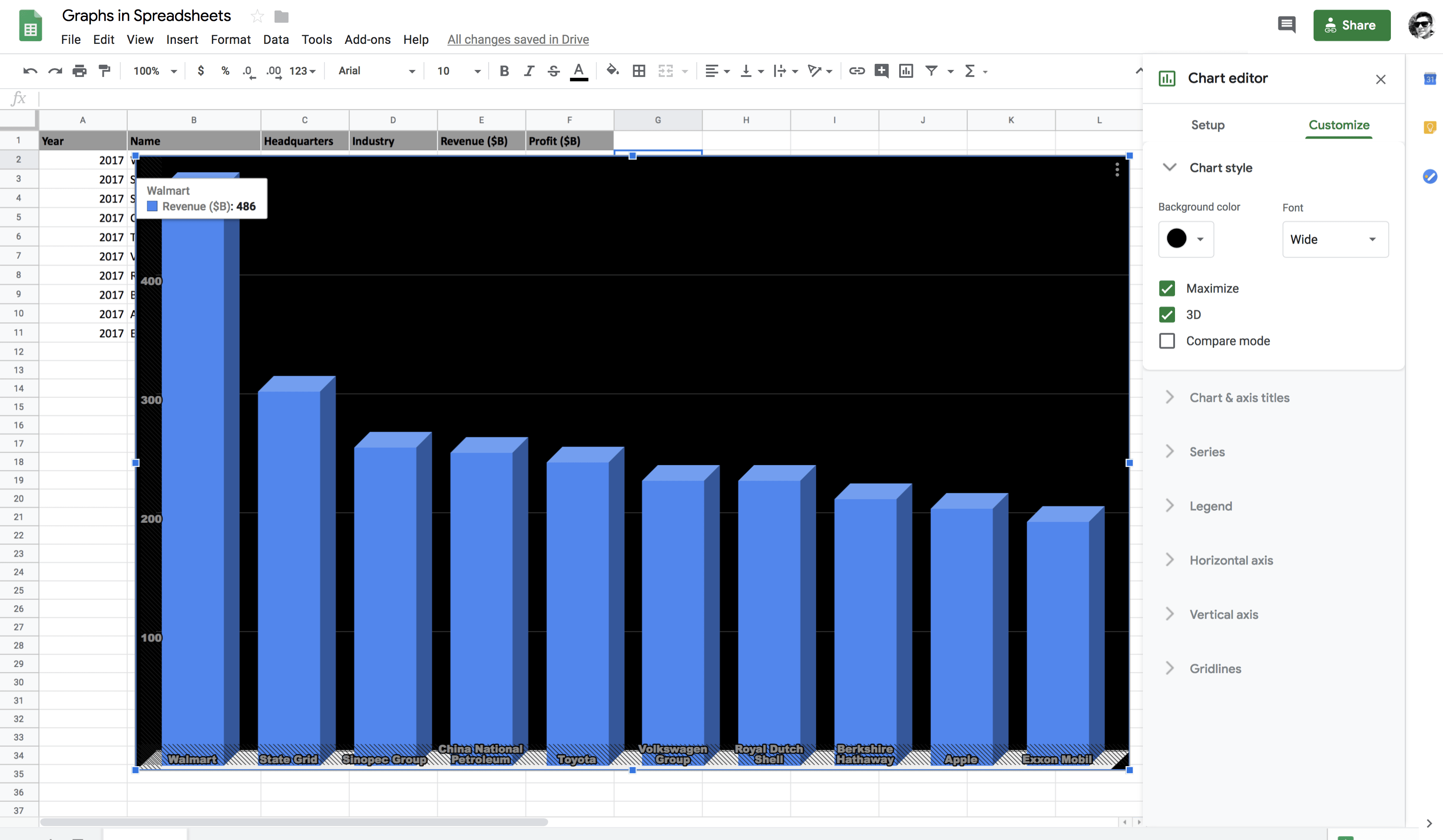• Subtract operation does not have a dedicated function to it, but it can be performed using the ADD and SUM functions by placing a - (minus) sign between the cells you would like to subtract.

Let's find out how you can do that in spreadsheets.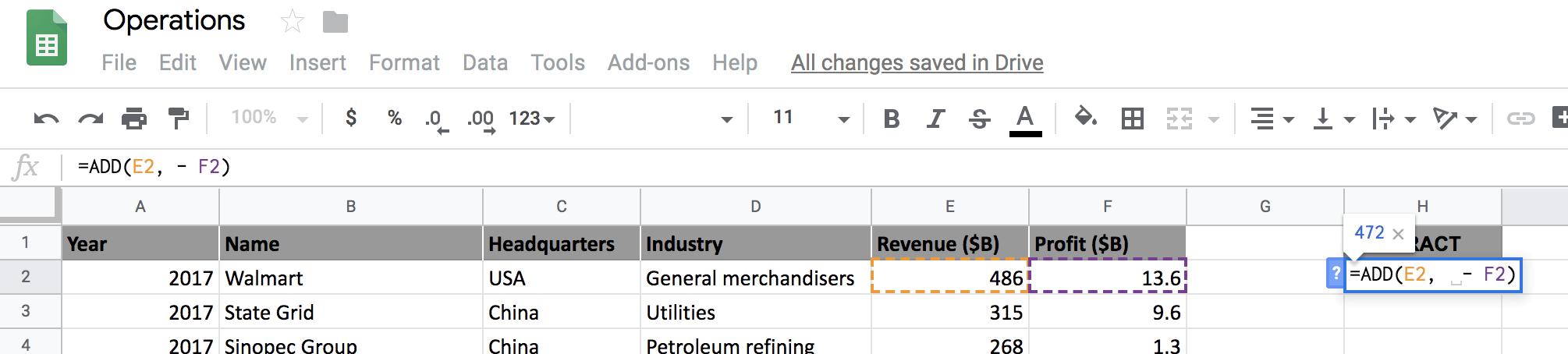As you can observe from the above figure that by just adding a - sign in place of a + you are now performing subtraction.

Now you will learn to multiply in spreadsheets!

• Multiplying is as simple as addition and subtraction. It can be done either by using a * (asterisk) sign or by using a PRODUCT function.

First, let's unroll the asterisk way and then you will also use the PRODUCT function to multiply.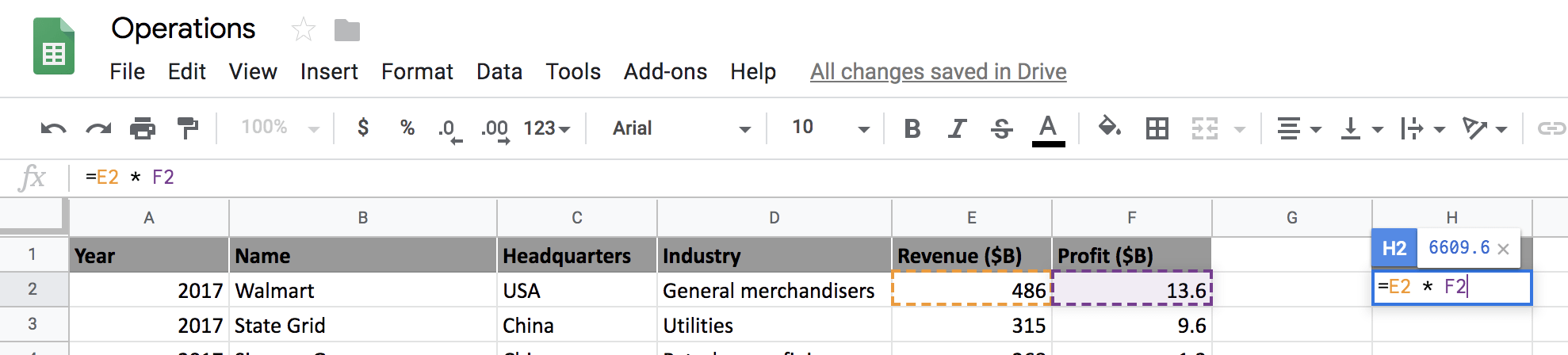Now let's select a range of cells from Profit and multiply them using the PRODUCT function. The formula will calculate the product of all the values in the range F2: F6.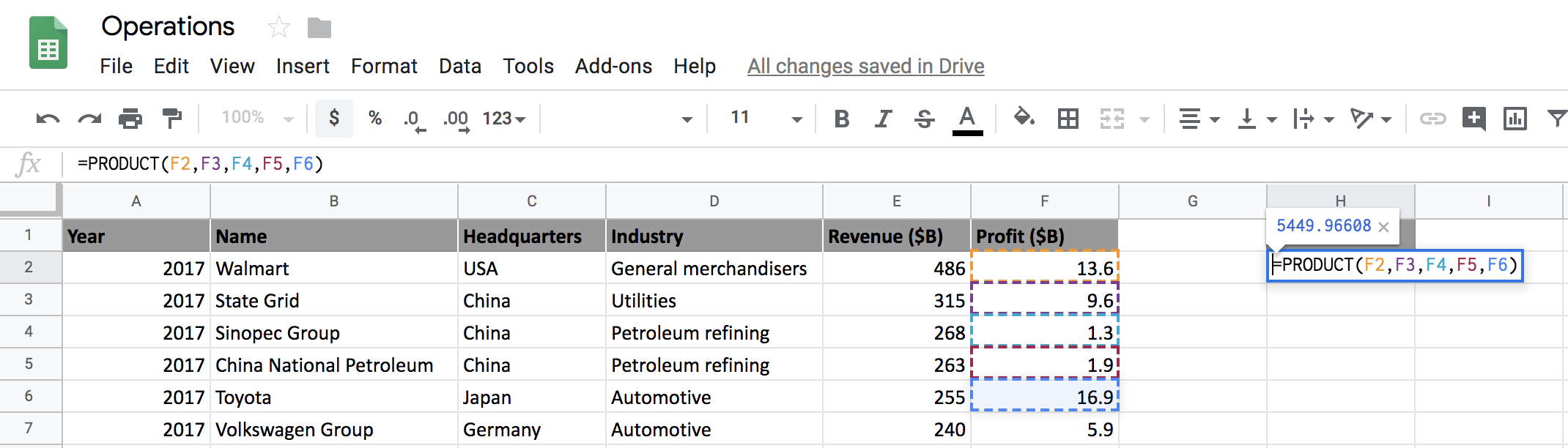• Division can be easily performed using a / (forward) slash in which the left of the forward slash is the dividend and to the right of the forward slash is the divisor.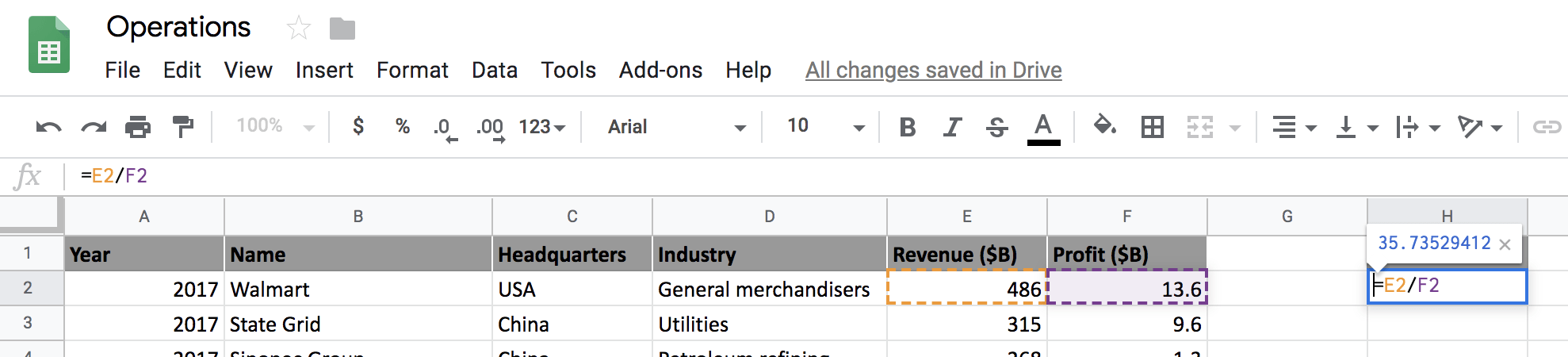Similarly, you can use a DIVIDE function to perform the division instead of using the forward slash as shown in the figure below.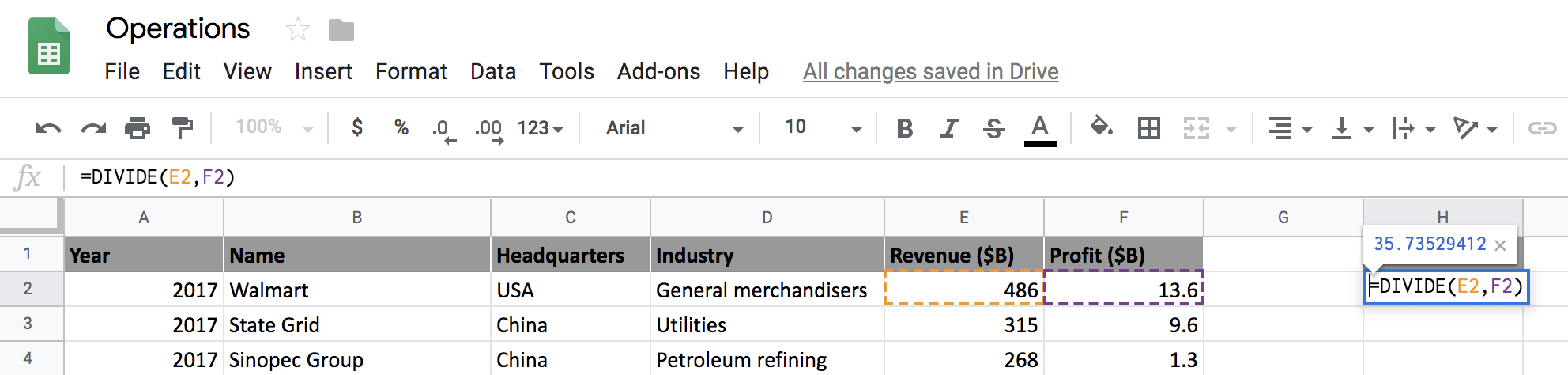What if the Divisor is zero?

If you divide a number by zero, you will get a divide by zero error. This means that the divisor can be any number but not zero.

Let's change the value of cell F2 to zero and divide the cell E2 by F2 and see what output you get.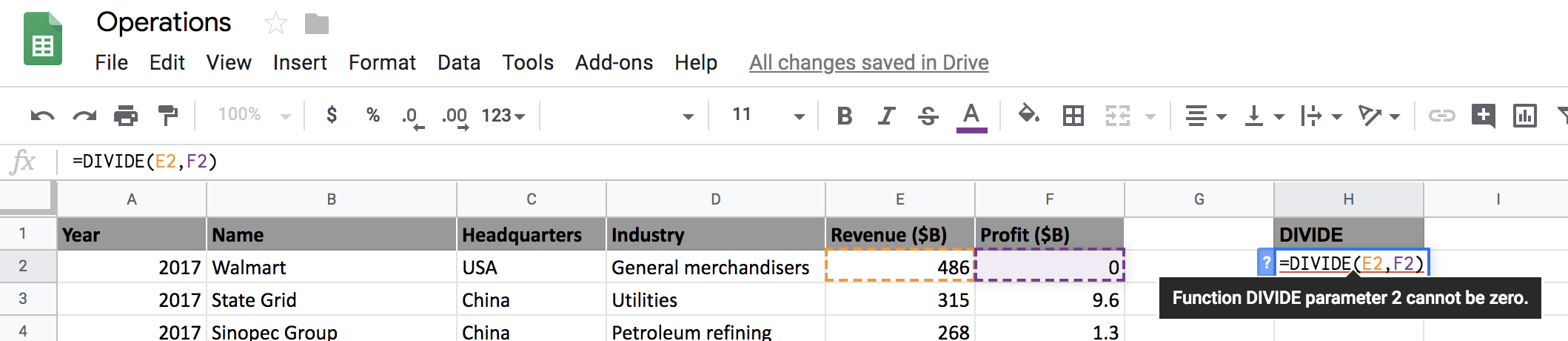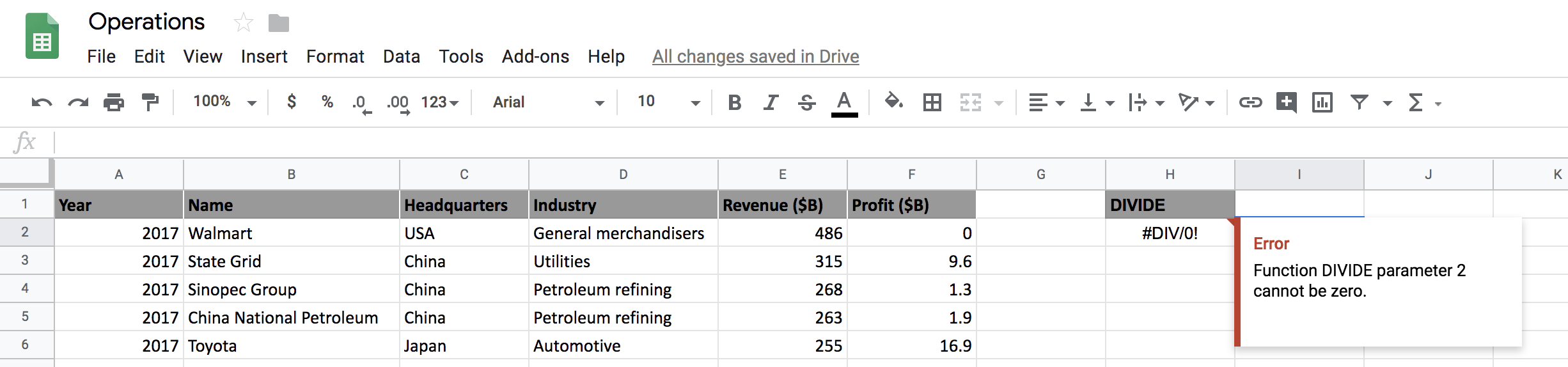From the above two figures, you can observe that the google spreadsheets throws an error Function DIVIDE parameter 2 cannot be zero since the divisor (cell F2) is zero.

Next, you will learn to use square roots in spreadsheets, so let's quickly start.

• Calculating Square Roots in spreadsheets is pretty straightforward. All you need to do is use the SQRT function which takes a single positive cell value as an input and returns a positive square root.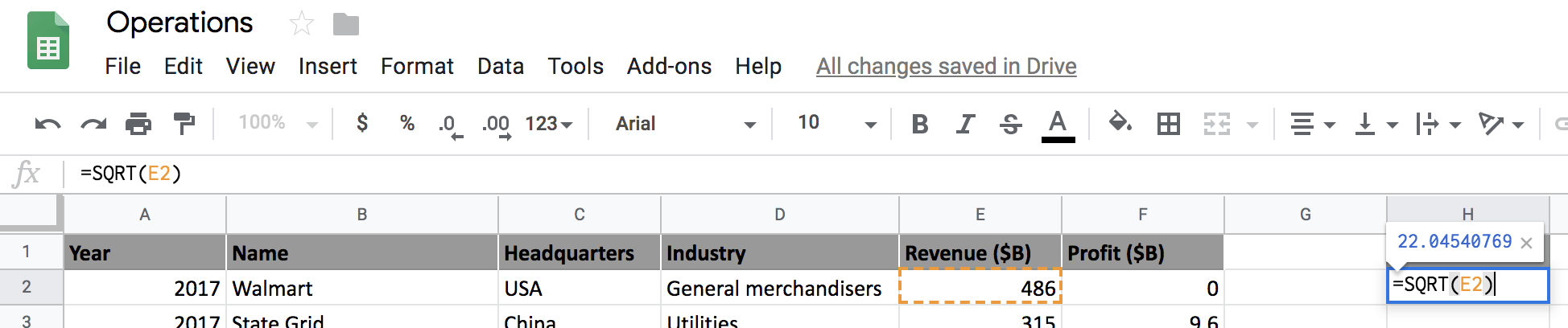What if the Revenue is Negative?

Even though the revenue cannot be negative, to understand the functionality of the SQRT function, let's assume that cell E2 has a value of -486 and try calculating the square root of it and see what output it gives.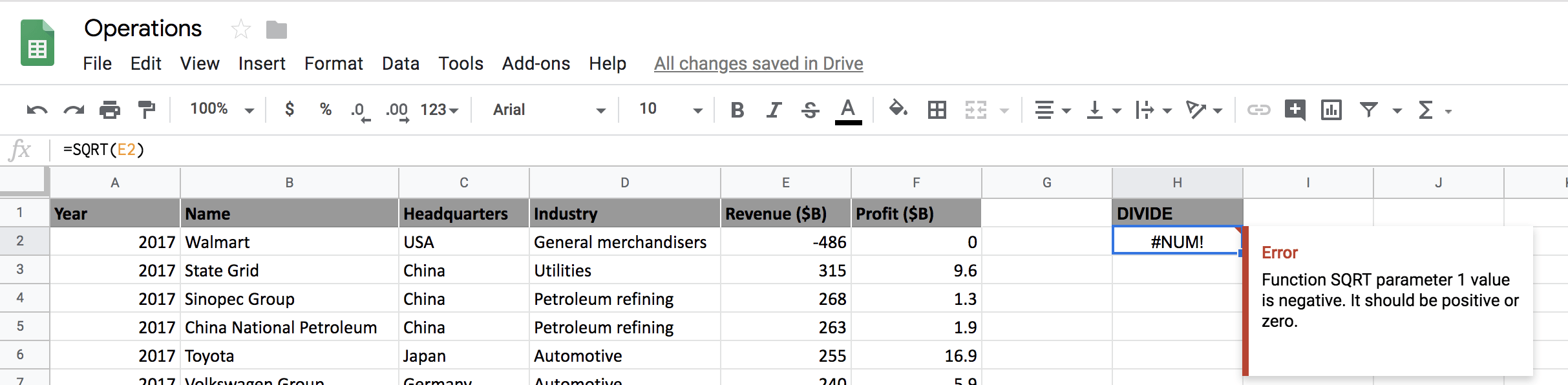As you can see from the above figure that the square root of a negative number can not be calculated in spreadsheets as it throws an error.

However, you have a workaround, which is by using the ABS function, which will return the absolute (positive) value of the number. Once you have a positive number, you can then apply the SQRT function.

The formula is given as =SQRT(ABS(E2)) which is a nested formula where the absolute value of E2 is first calculated and on that absolute value the square root function is applied.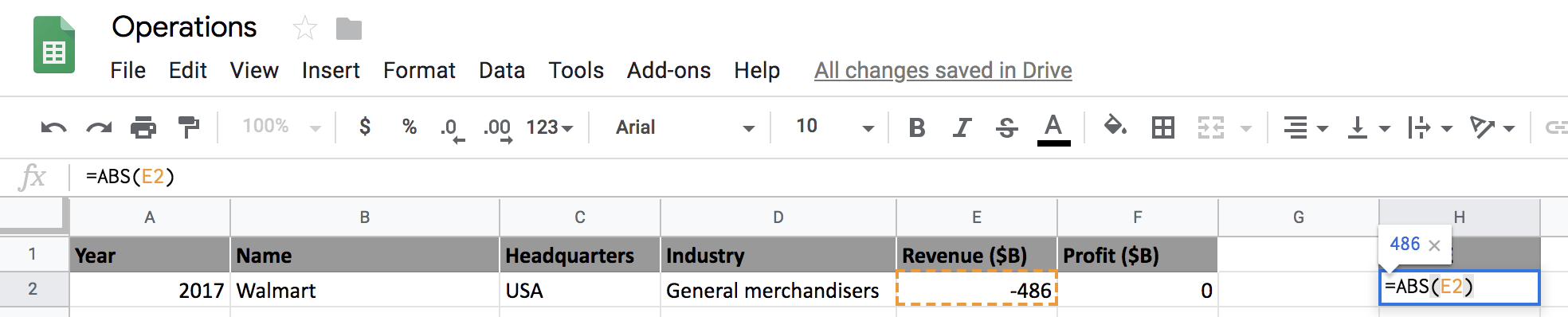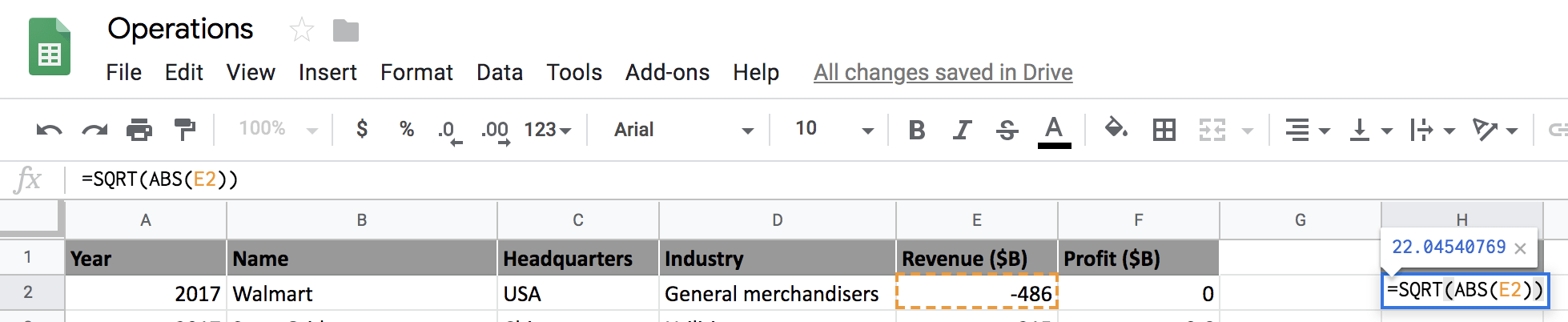In the next and final segment of the tutorial, you will learn about exponents.

• Exponents are very simple to use and can be used in google spreadsheets in two ways either with a ^ (caret) sign or with an in-built POWER function.

POWER function takes in two parameters in which the first parameter is the base and the second parameter is the exponent. It returns a number raised to a power. The base is a number to raise to the exponent power, and the exponent is the power to raise the base to.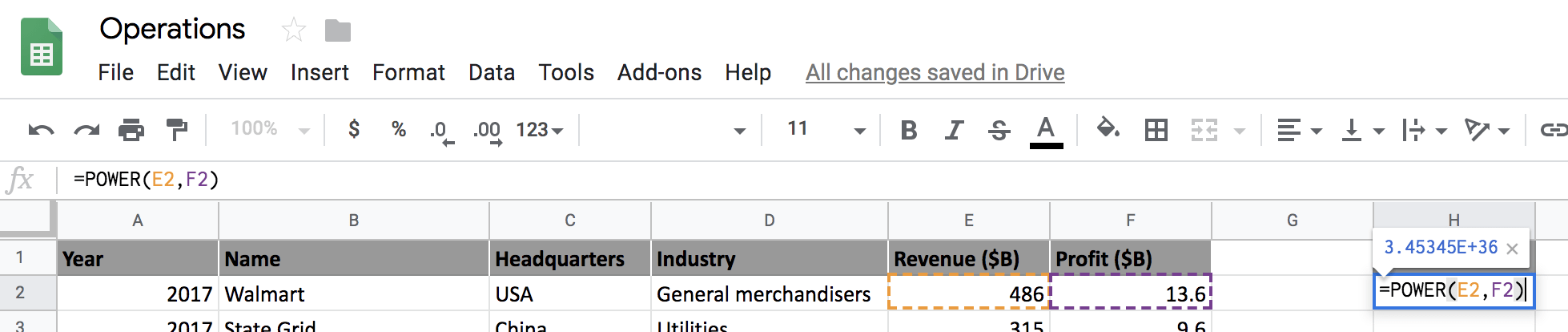In the above figure, you can see that raising Revenue, having a value of 486 to a Profit of 13.6, results in a huge number.

Go Further!

Congratulations on finishing the tutorial.

This tutorial was an excellent introduction to Mathematical Operations in Spreadsheets which covered most of the components of mathematical operations.

A small exercise that would take you one step closer to mastering this topic is by combining all the mathematical operations discussed in this tutorial together and creating a custom formula which should follow the PEMDAS rule.